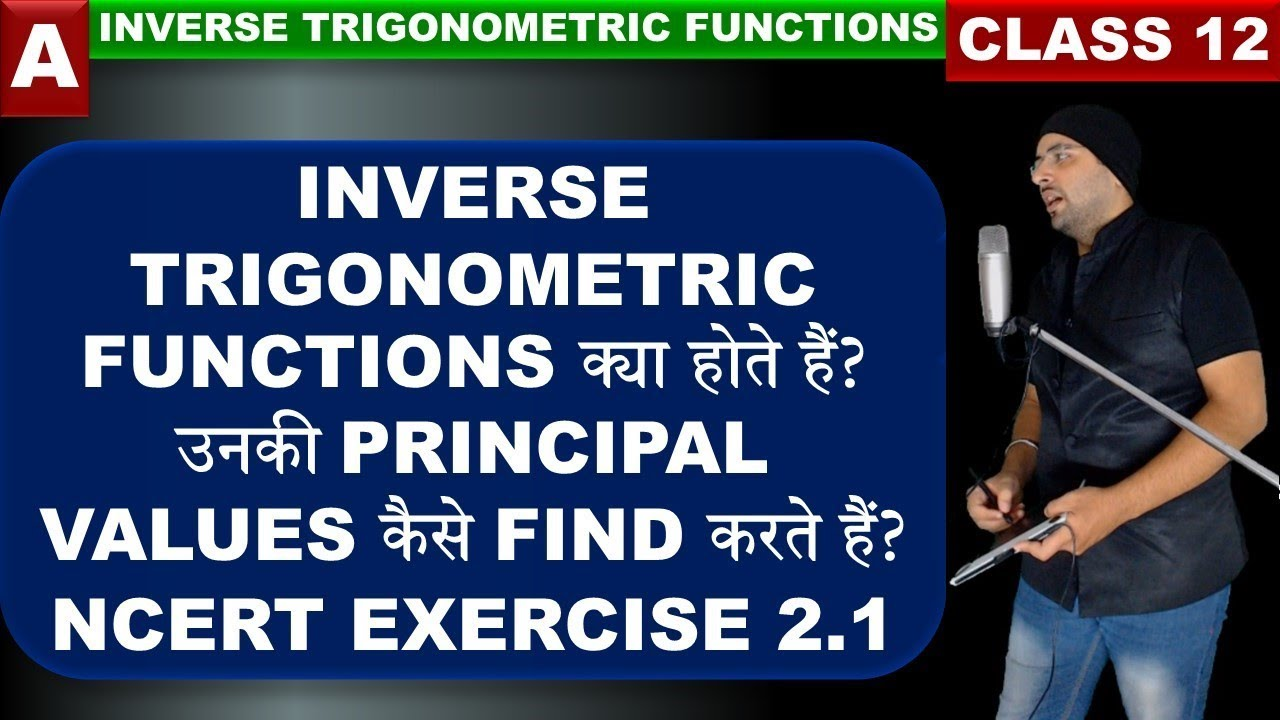## Exercise 2.1 Inverse Trigonometric Functions

Topics/Questions discussed in this video: 00:00:30 What is the meaning of Inverse Trigonometry 00:03:40 Conditions when inverse of a function exists 00:17:50 Range of Inverse trigonometric functions (Principal values) 00:21:13 Derivation for negative input in inverse...## Exercise 1.1 Relations and Functions Class 12 Maths Chapter 1

Topics/Questions discussed in this video: Question 1. Let R be the relation in the set N given by R = {(a, b) : a = b – 2, b in 6}. Choose the correct answer. A. (2, 4) ∈ R B. (3, 8) ∈ R C. (6, 8) ∈ R D. (8, 7) ∈ R (N) Question 2. Let A= {1, 2, 3,} and define R = {(a,...## Exercise 2.2 Inverse Trigonometric Functions Class 12 Maths

Topics/Questions discussed in this video: 00:00:00 Introduction for inverse trigonometry identities 00:03:34 Derivation of all inverse trigonometric identities Prove the following: 00:15:14 NCERT Solutions Exercise 2.2 Question 1 [latex]3{sin ^{ - 1}}x = {sin ^{ -...## Exercise 6.2 Application of Derivatives Class 12 Maths Chapter 6

Topics/Questions discussed in this video: In this lecture, I am discussing how to find intervals in which the given function is increasing and decreasing. Also, questions based on this topic from NCERT Exercise 6.2 Questions discussed in this lecture: NCERT EXERCISE...